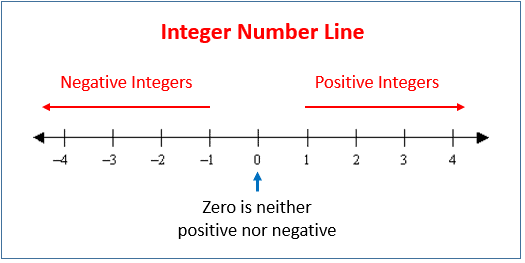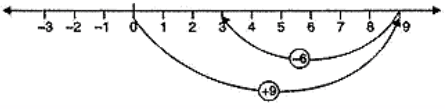Courses

# Chapter Notes - Integers Class 6 Notes | EduRev

## Class 6 : Chapter Notes - Integers Class 6 Notes | EduRev

The document Chapter Notes - Integers Class 6 Notes | EduRev is a part of the Class 6 Course Mathematics (Maths) Class 6.
All you need of Class 6 at this link: Class 6

Integers

If you move towards the right from the zero mark on the number line, the value of the numbers increases. If you move towards the left from the zero mark on the number line, the value of the numbers decreases.The collection of the numbers, that is, … -3, -2, -1, 0, 1, 2, 3, …., is called integers

When we need to use numbers with a negative sign, we need to go to the left of zero on the number line. These numbers are called negative numbers.

Examples where these negative numbers are used are temperature scale, water level in a lake or river, level of oil tank, debit account and outstanding dues.

The numbers -1, -2, -3, -4… which are called negative numbers, are also called negative integers.

The number 1, 2, 3, 4 …s, which are called positive numbers, are also called positive integers.

If we stand at the zero mark on the number line, we can either go left towards negative integers or right towards positive integers. When we move left towards zero on the number line, the value of positive integers decreases. When we move left further away from zero on the number line, the value of negative integers decreases.

When two positive integers are added, then we get an integer with a positive sign.

Example: (+8) + (+6)= + 14

When two negative integers are added, then we get an integer with a negative sign.

Example: (-3) + (-5) = -8

When a positive integer is added to a negative integer, then we subtract them and put the sign of the greater integer. The greater integer can be decided by ignoring the signs of the integers. Example: (+4) + (-9) = -5; (+8) + (-3) = 5

Subtraction of integers:

When we subtract a larger positive integer from a smaller positive integer, the difference is a negative integer.

Example: (+5)-(+8) = -3

To subtract a negative integer from any given integer, we just add the additive inverse of the negative integer to the given integer.

Example: (-5)-(-8) = +3

Thus, the subtraction of an integer is the same as the addition of its additive inverse. Both addition and subtraction of integers can be shown on a number line.

PRACTICE QUESTIONS
Q.1. Write all the integers between -8 and -15. (Write them in the increasing order.)
Ans. The integers between –8 and –15 in increasing order are –14, –13, –12, –11, –10 and –9.

Q.2. Find the solution of the following :(–9) + (+13)
Ans. (–9) + (+13)
= (–9) + (+9) + (+4)
= 0 + (+4) = +4

Q.3. Subtract :(–20) – (–13)
Ans. (–20) – (–13)
= (–20) + (additive inverse of -13)
= (–20) + (13) = –7

Q.4. Find the value of :(–7) + (–9) + 4 + 16
Ans. (–7) + (–9) + 4 + 16
= (–16) + 20
= (–16) + 16 + 4
= 0 + 4 = 4

Q.5. Using number line, add the following integers: 9 + (-6).
Ans. On the number line we first move 9 steps to the right from 0 reaching 9 and then we move 6 steps to the left of 9 and reach 3.
Thus, 9 + (-6) = 3Q.6. The temperature on a certain morning is -11℃ at 5 a. m. If the temperature drops 3 degree at 6 a.m. and rises 5 degree at 8 a.m. and again drops 3 degree at 9 a.m. What is the temperature at 9 a.m.?
Ans. Temperature at 5 a.m. = -11℃
Temperature decreased at 6 a.m. = 3℃ = -3
Temperature raised at 8 a.m. = 5℃ = +5
Temperature decreased at 9 a.m. = 3℃ = -3
Final temperature at 9 a.m. = (-11) + (-3) + (+5) + (-3)
= -11 – 3 + 5 – 3
= -17 + 5
= -12℃.

Offer running on EduRev: Apply code STAYHOME200 to get INR 200 off on our premium plan EduRev Infinity!

## Mathematics (Maths) Class 6

191 videos|220 docs|43 tests

,

,

,

,

,

,

,

,

,

,

,

,

,

,

,

,

,

,

,

,

,

;